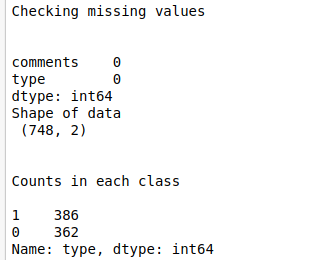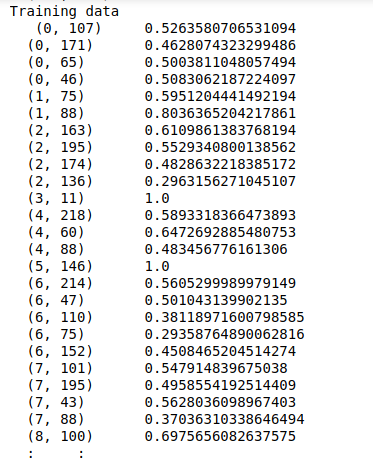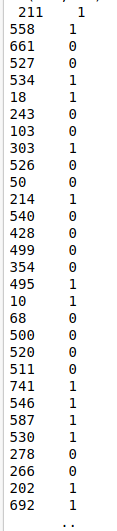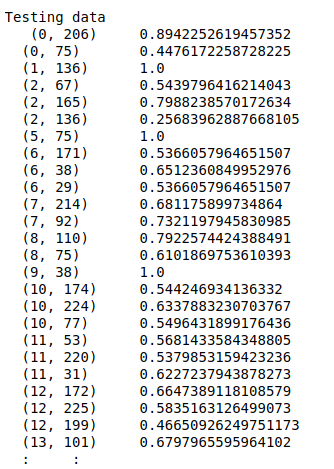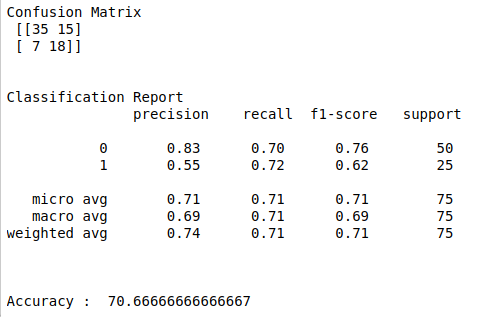• #5, First Floor, 4th Street Dr. Subbarayan Nagar Kodambakkam, Chennai-600 024 Landmark : Samiyar Madam
• pro@slogix.in
• +91- 81240 01111

### Movie review sentimental analysis using keras in python?

###### Description

To build a deep learning model for classify movie reviews using keras.

###### Input

Imdb movie review data set (Kaggle).

###### Output

Confusion matrix, classification report and accuracy score.

#### process:

Load the data set.

Do pre-pocessing like remove punctuation, stopwords, etc..

Convert text data into vector format.

Define the independent and dependent variable.

Build the deep neural networks.

Initiate activation and optimizer functions according to the problem.

Compile and fit the DNN model.

Split the data into train and testing set.

Fit the training set into the model.

Predict the test results using DNN.

Calculate the accuracy, precision and recall.

###### Sample Code

#import necessary libraries
import warnings
warnings.filterwarnings(“ignore”)
import pandas as pd
import numpy as np
import time
import re
from sklearn.feature_extraction.text import TfidfVectorizer
import matplotlib.pyplot as plt
import seaborn as sns
from keras.models import Sequential
from keras.layers import Dense
from sklearn.model_selection import train_test_split
from keras.utils import np_utils
from sklearn.metrics import classification_report, confusion_matrix

data = pd.read_table(‘/home/soft50/soft50/Sathish/practice/imdb_labelled.txt’,sep=”\t”,names = names)

#check missing values
print(“Checking missing values\n\n”)
print(data.isnull().sum())

#make it as a data frame
df = pd.DataFrame(data)

#print data shape
print(“Shape of data\n”,df.shape)

#counts in each class
print(“\n”)
print(“Counts in each class\n”)
print(df[‘type’].value_counts())

#Features
y = df[‘type’]

#change text lower cases and removal of white spaces
lower_text = []
for i in range(0,len(X)):
s = str(X[i])
s1 = s.strip()
lower_text.append(s1.lower())
#print(“After converting text to lower case\n\n”,lower_text)

#Remove punctuation
punc_text = []
for i in range(0,len(lower_text)):
s2 = (lower_text[i])
s3 = re.sub(r'[^\w\s2]’,”,s2)
punc_text.append(s3)
#print(“After removed punctuation\n\n”,punc_text)

#Word vectorization
#Initialize the TF-IDF vectorizer
tfidf = TfidfVectorizer(sublinear_tf=True, min_df=5,max_df = 0.7,norm=’l2′, encoding=’latin-1′, ngram_range=(1, 2),
stop_words=’english’)

#transform independent variable using TF-IDF vectorizer
X_tfidf = tfidf.fit_transform(punc_text)
print(“After vectorized text data\n\n”,X_tfidf)

#Split the data into train and testing
X_train, X_test, Y_train, Y_test = train_test_split(X_tfidf, y, test_size=0.1, random_state=0)

#Print training data
print(“Training data\n”,X_train,”\n”,Y_train)
print(“\n\n”)

#Print testing data
print(“Testing data\n”,X_test)
print(“\n\n”)

#make dependent variable categorical
Y_train = np_utils.to_categorical(Y_train,num_classes=2)
Y_test = np_utils.to_categorical(Y_test,num_classes=2)

batch_size = 20

#shape of input
n_cols_2 = X_train.shape

#create deep neural networks
model_2 = Sequential()

#add layers to model
print(model_2.summary())

#Compile the model

#Here we train the Network.
start_time = time.time()
model_2.fit(X_train, Y_train, batch_size = batch_size, epochs = 10, verbose = 2)
end_time = time.time()
elapsed_time = end_time – start_time
print(“Time to train model: %.3f seconds” % elapsed_time)

#Evaluate the network
start_time = time.time()
score,acc = model_2.evaluate(X_test,Y_test,verbose = 2,batch_size = batch_size)
end_time = time.time()
elapsed_time = end_time – start_time
print(“Time to evaluate model: %.3f seconds” % elapsed_time)
print(“\n”)

#Predict the test results
prediction = model_2.predict(X_test)
length = len(prediction)
y_label = np.argmax(Y_test,axis=1)
predict_label = np.argmax(prediction,axis=1)

#classification report
print(“Confusion Matrix\n”,confusion_matrix(Y_test.argmax(axis=1),prediction.argmax(axis=1)))
print(“\n”)
print(“Classification Report\n”,classification_report(Y_test.argmax(axis=1),prediction.argmax(axis=1)))
print(“\n”)
accuracy = np.sum(y_label==predict_label)/length * 100
print(“Accuracy : “,accuracy)

###### Screenshots